# Searching a String for Matching Word from another String in Microsoft Excel 2010

To search a string for a matching word from another string, we use the "IF", "ISNUMBER", "FIND" and "LEFT" functions in Microsoft Excel 2010.

IF: - Checks whether a condition is met and returns one value if True and another value if False.

Syntax of “IF” function =if(logical test,[value_if_true],[value_if_false])

The logical test is performed and if true, the value_if_true output is given, else the output in the value_if_false parameter is given.

For example:Cells A2 and A3 contain the numbers 3 and 5. If the number in the cell is 3, then the formula should display “Yes”, else “No”.

=IF (A1=3,"Yes","No")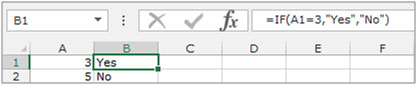ISNUMBER: - In Microsoft Excel, “ISNUMBER” function is used to check if the value in the cell contains a number or not.

Syntax of “ISNUMBER” function: =ISNUMBER (value)

Example:Cell A2 contains the number456

=ISNUMBER (A2), function will return true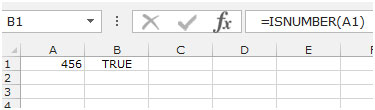FIND:This function returns the location number of the character at which a specific character or text string is first found, reading left to right (not case-sensitive).

Syntax of “FIND” function:           =FIND (find_text,within_text,[start_num])

Example:Cell A1contains the text “Broncho Billy Anderson”

=FIND ("Billy", A1, 1), function will return 9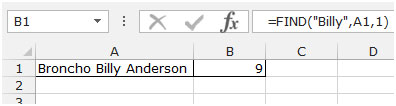LEFT: Returns the specified number of characters starting from the left-most character in the string.

Syntax of “LEFT” function:            =LEFT (text,[num_chars])

Example:Cell A1contains the text “Broncho Billy Anderson”

=LEFT (A1, 7), function will return “Broncho”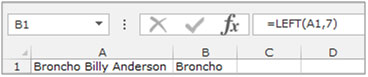Let’s take an example to understand how we can search the string for a matching word from another string.

Example 1: We have 2 lists,in column A and column B. Weneed to match the first word in each cell in column A with column B.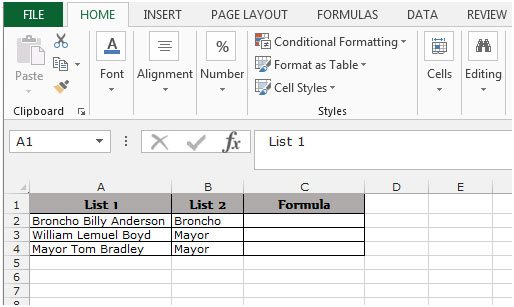• Select the cell C2, write the formula
• Press Enter on your keyboard.
• The function will search a string for a matching word from another string. It will compare the string in List 2 with List 1 and if found, it will return “1st Word Found”, else it will return “1st Word Not Found”.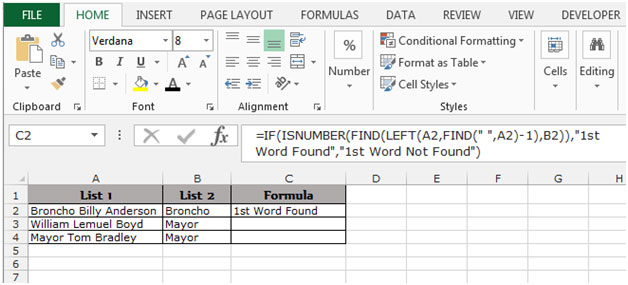• To copy the formula in all cells, press the key “CTRL + C” and select the cell C3:C4 and press the key “CTRL + V” on your keyboard.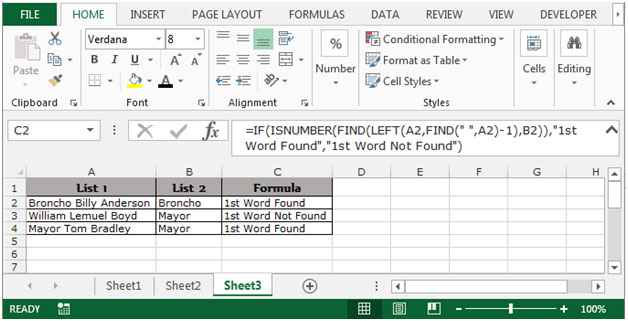This is how we can search a string for a matching word from another string in Microsoft Excel.

Terms and Conditions of use

The applications/code on this site are distributed as is and without warranties or liability. In no event shall the owner of the copyrights, or the authors of the applications/code be liable for any loss of profit, any problems or any damage resulting from the use or evaluation of the applications/code.